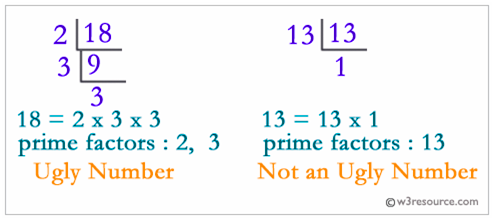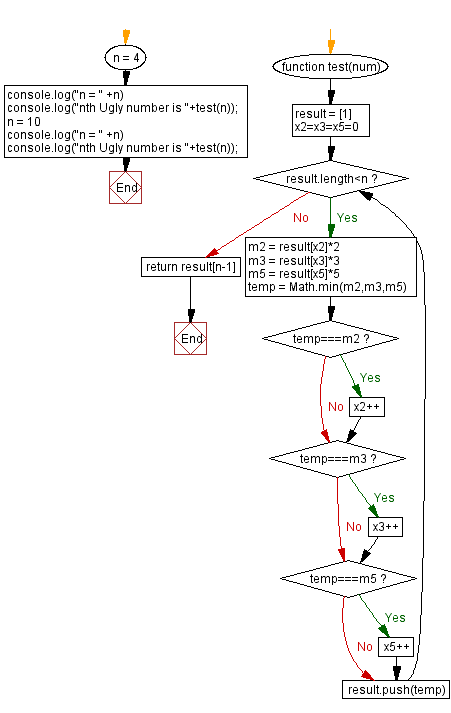# JavaScript: Find the nth ugly number

## JavaScript Math: Exercise-101 with Solution

Write a JavaScript program to find the nth ugly number.

Ugly numbers are positive numbers whose only prime factors are 2, 3 or 5. The first 10 ugly numbers are:
1, 2, 3, 4, 5, 6, 8, 9, 10, 12, ...
Note: 1 is typically treated as an ugly number.

Visualisation:Test Data:
(4) -> 4
(10) -> 12

Sample Solution:

HTML Code:

``````<!DOCTYPE html>
<html>
<meta charset="utf-8">
<title>JavaScript program to Find the nth ugly number</title>
<body>

</body>
</html>
```
```

JavaScript Code:

``````function test(num) {
result = 
x2=x3=x5=0
while (result.length<n){
m2 = result[x2]*2
m3 = result[x3]*3
m5 = result[x5]*5
temp = Math.min(m2,m3,m5)
if(temp===m2){
x2++
}
if(temp===m3){
x3++
}
if(temp===m5){
x5++
}
result.push(temp)
}
return result[n-1]
};
n = 4
console.log("n = " +n)
console.log("nth Ugly number is "+test(n));
n = 10
console.log("n = " +n)
console.log("nth Ugly number is "+test(n));
```
```

Sample Output:

```n = 4
nth Ugly number is 4
n = 10
nth Ugly number is 12
```

Flowchart:Live Demo:

See the Pen javascript-math-exercise-101 by w3resource (@w3resource) on CodePen.

Improve this sample solution and post your code through Disqus

What is the difficulty level of this exercise?

Test your Programming skills with w3resource's quiz.

﻿